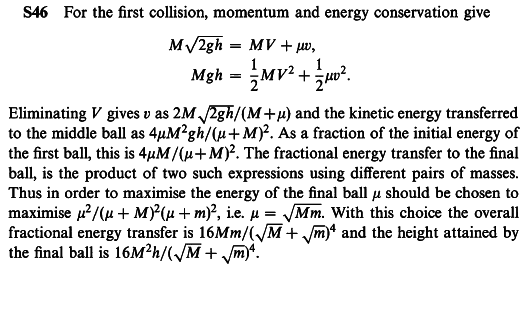# Newton's cradle height problem

## Homework Statement

An executive toy consists of three suspended steel balls of masses M, n and m arranged in order with their centres in a horizontal line. The ball of mass M is drawn aside in their common plane until its centre has been raised by h and is then released. if M ≠ m and all collisions are elastic, how must n be chosen so that the ball of mass m rises to the gratest possible height? What is that height? (Neglect multiple collisions)

## Homework Equations

velocity after a perfectly elastic collision
v2' = (m2 - m1)v2/(m1+m2) + 2m1v1/(m1+m2)

## The Attempt at a Solution

After releasing M, its vellocity immediately before the first collision is
$V = \sqrt{2gh}$

Then, M collides with n, and n's velocity immediately after collision is
$v = \frac{2M}{M+n}\sqrt{2gh}$

Analogously, m's velocity immediately after n colides
$u = \frac{2n}{n+m}\frac{2M}{M+n}\sqrt{2gh}$

m shall rises H
$mu^{2}/2 = mgH$

$H = 16h\frac{M^{2}n^{2}}{(M+n)^{2}(m+n)^{2}}$

How am I supposed to maximize H from it? What should be done to solve it?

Last edited:

## Answers and Replies

TSny
Homework Helper
Gold Member
Looks good so far. You have found H as a function of n. Are you familiar with using calculus to find the maximum of a function?

But if you derivate with respect to n, you get
2n³ + (M+m-1)n² - (M+m)n - Mm = 0
probably, three roots, one of them might get H to max. The problem is to find these roots.
By the way, this problem is from a test which you should take 8 minutes each question: there must be a better way to solve it.

Last edited:
I've found a solutionWhat sorcery did it do to get n = √(Mm)??

TSny
Homework Helper
Gold Member
Note that the equation $H = 16h\frac{M^{2}n^{2}}{(M+n)^{2}(m+n)^{2}}$ can be written as $$H = 16hM^2\left(\frac{n}{(M+n)(m+n)}\right)^2$$

H will be maximum when the expression ##\frac{n}{(M+n)(m+n)}## is a maximum. So, you just need to maximize the function $$f(n) = \frac{n}{(M+n)(m+n)}$$

Oh, thanks, TSny, I didn't see the reply. I got it